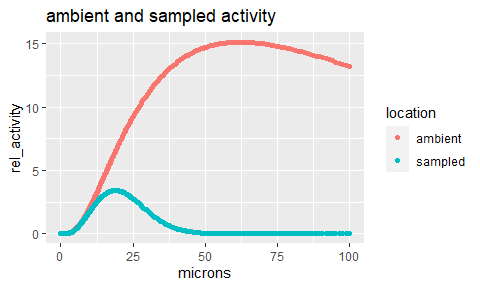# Using AeroSampleR to model aerosol sampling efficiency

## AeroSampleR Introduction

Air sampling systems are used in many applications that require the monitoring of hazardous airborne particles. When tubing is used to collect aerosol particles, some of the particles are lost along the way. The efficiency of the system depends on the particle and tubing configuration.

This version of AeroSampleR provides sampling efficiency for a limited set of system elements. Sampling systems always include a probe, after which are combinations of straight tubing and bends. While some systems include expansion or contraction elements, or sample splitters. These components are not covered in this version of AeroSampleR. The probe model is limited to a simple open ended pipe in still air.

AeroSampleR relies on the concept of aerodynamic median activity diameter (AMAD), which accounts for particle density and shape, leaving equivalent spherical water droplets as the modeling targets.

Efficiency functions are based predominantly on testing with aerosol particles through stainless steel tubing. The Zhang, McFarland, and Pui bend models are used in this package.

The aerosol transport models are based on tests on clean systems. This package is designed primarily for new tubing designs. If a system is not maintained clean – and free of condensation, there can be no expectation that sampling efficiency models will be accurate.

### example data

The first task in evaluating a system is to set up a table that includes all the “elements” with the following column headers:

• el_num sequential number of the element
• el_type starting with “probe”, followed by “tube” and “bend” elements
• length_cm length of tubes in centimeters. Leave blank for probe and bends.
• angle_to_horiz degree of slope of straight tube elements. Leave blank for probes and bends.
• orient orientation of the probe. Options are “u” for up, “d” for down and “h” for horizontal bend_angle how many degrees a bend turns the sample. Typically, 90 or 45. Leave blank for probes and tubes.
• bend_rad_cm the bend radius in centimeters. Leave blank for probes and tubes.

el_num

el_type

length_cm

angle_to_horiz

orient

bend_angle

1

probe

u

2

tube

112

90

3

bend

90

13

4

tube

146

0

### 1) Get the system data

You need to get this data into R and call it sys_df, the “system” data frame. There are many options on how to do this. Below are two examples. You will have to provide the path and the file name, but for the example, we’ll show the file to be called system.txt or system.xlsx in c:/work. Here are two:

1. Use base R, (utils package that is loaded with base R) to read a text file:
sys_df <- read.table(
file = "c:/work/system.txt",
)
sys_df <- readxl::read_xlsx(path = "c:/work/system.xlsx",
sheet = "Sheet1", #default - update if needed
range = "A1:G5", #put in entire range
col_types = c("numeric",
"text",
"numeric",
"numeric",
"text",
"numeric",
"numeric")
)

### 2) Create particle distribution with particle_dist()

This function provides a logarithmic distribution of 1000 particle sizes and an additional set of discrete particles. By default, the logarithmically-distributed particles have an AMAD of 5 and a lognormal standard deviation of 2.5, consistent with ICRP 66.

The discrete particles are 1, 5, and 10 micrometers AMAD.

df <- particle_dist() #Default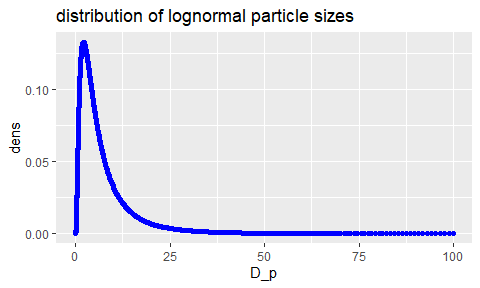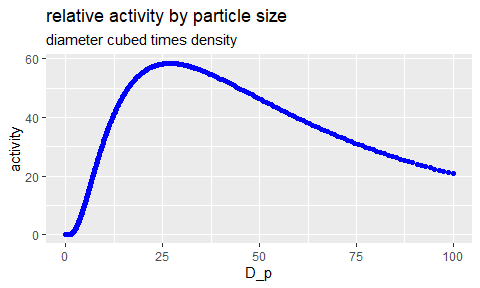### 3) Set up the parameters for tube size, flow rate, temperature, and pressure. These parameters are not particle dependent and so can be kept in a small separate data frame.

• D_tube is the inner diameter of the tube {cm}
• Q_lpm is the flow rate of air {lpm}
• T_C is the system pressure {Celsius}
• P_kPa is the pressure of the system {kPa}
# In this example the tubing wall is 1.65 mm thick.
params <- set_params_1("D_tube" = 2.54 - (2 * 0.165), #1 inch tube diameter
"Q_lpm" = 2 * 28.3, #2 cfm converted to lpm
"T_C" = 25,
"P_kPa" = 101.325)

Next, we compute the particle size-dependent parameters. These include factors for transport efficiency computation. - Cunningham Correction Factor {C_c} - terminal settling velocity {v_ts} - Particle Reynolds number (tube) {Re_p} - Stokes number {Stk}

df <- set_params_2(df, params)

At this point, our main particle distribution data frame has been modified with computed factors for use in the transport efficiency models, row by row.

### 4) Next, we compute the efficiency, element by element in transport order. We have only four elements in this example and we will evaluate them with prob_eff(), tube_eff(), bend_eff(), and lastly tube_eff() again. This will add columns to our particle data frame.

Calculate the efficiency of the probe via prob_eff() and add it to a new data frame. The orient argument sets the orientation of the probe. “u” means the probe is vertically upward. “d” is for a vertically downward facing probe. “h” is for a probe in a side configuration. The probe is in the first row, so we use  to identify the orient parameter.

df <- probe_eff(df, params, orient = sys_df$orient)  Calculate the efficiency of the first tube. Tube Efficiency is found using tube_eff() function. The length is given in cm (length_cm) and the angle from tube to horizontal orientation parameter (angle_to_horiz) is specified here. All three parameters can be added to the above data frame, which will return a column for each distribution. df <- tube_eff(df, params, L = sys_df$length_cm / 100,
angle_to_horiz = sys_df$angle_to_horiz, elnum = sys_df$el_num)

Calculate the efficiency of the bend. Here, we’ll take the Zhang model option. Bend Efficiency is found via the bend_eff() function and is where you will choose to use one of three different tube models {Zhang, McFarland, or Pui}. The bend angle and element number are also listed in the function.

df <- bend_eff(df, params, method = "Zhang",
bend_angle = sys_df$bend_angle, bend_radius = sys_df$bend_rad_cm / 100,
elnum = sys_df$el_num) Finally, we’ll calculate transport efficiency through the last tube element. df <- tube_eff(df, params, L = sys_df$length_cm / 100,
angle_to_horiz = sys_df$angle_to_horiz, elnum = sys_df$el_num)

### At this point, the transport efficiencies have been built into the data frame. Let’s have a look at the bottom few rows.

It doesn’t all fit horizontally, so match the top portion and the bottom portion by the row number.

tail(df)
#>            D_p         dens     dist      C_c         v_ts         Re_p
#> 998   97.58596 2.323286e-05 log_norm 1.001598 2.828305e-01 1.778384e+00
#> 999   98.78560 2.197752e-05 log_norm 1.001579 2.898214e-01 1.844744e+00
#> 1000 100.00000 2.078631e-05 log_norm 1.001560 2.969852e-01 1.913581e+00
#> 1001   1.00000 1.000000e+00 discrete 1.203362 3.568242e-05 2.299144e-06
#> 1002   5.00000 1.000000e+00 discrete 1.033187 7.659083e-04 2.467509e-04
#> 1003  10.00000 1.000000e+00 discrete 1.015739 3.011898e-03 1.940672e-03
#>               Stk eff_probe eff_tube_2   eff_bend_3 eff_tube_4
#> 998  6.4182782401 0.0000000  0.9999999 1.593206e-09 0.04400467
#> 999  6.5769235956 0.0000000  0.9999999 9.356587e-10 0.03483797
#> 1000 6.7394918899 0.0000000  0.9999999 5.418602e-10 0.02709797
#> 1001 0.0008097418 1.0000000  0.9999987 9.985385e-01 0.99998936
#> 1002 0.0173807723 0.9999664  0.9999996 9.627252e-01 0.99979895
#> 1003 0.0683490543 0.9990397  0.9999998 8.498841e-01 0.99920750

### 5) Generate reports with report_basic, report_plots and report_cum_plots

The report_basic function provides total system efficiency for either all of the logarithmically distributed particles or all of the discrete particle sizes.

The report_plots function shows individual element efficiency. The report_cum_plots function shows cumulative efficiency through the system. This plot takes efficiency data from the rows of the data frame. It therefore only works for individually selected particle sizes.

We’ll show the parameter set first, so that the output message on the basic report, regarding units, makes sense.

params[, 7] <- formatC(params[, 7], digits = 2, format = "e")
params[, 8] <- formatC(params[, 8], digits = 2, format = "e")
params[, 11] <- formatC(params[, 11], digits = 2, format = "e")
params[, 3] <- formatC(params[, 3], digits = 4)
params[, 10] <- formatC(params[, 10], digits = 4)
ft <- flextable(params)
ft <- set_caption(ft, "system parameters")
ft
system parameters

D_tube

Q_lpm

velocity_air

T_K

P_kPa

density_air

viscosity_air

mfp

density_par

Re

k

0.0221

56.6

2.459

298.15

101.325

1.183907

1.84e-05

6.67e-02

1,000

3502

1.38e-23


ft <- flextable(report_basic(df, params, "discrete"))
#> System Parameters
#> All values in MKS units, except noted
#> Notes: D_tube is in m.
#> Q_lpm is system flow in liters per minute.
#> velocity_air is the derived air flow velocity in meters per second.T_K is system temperature in Kelvin.
#> P_kPa is system pressure in kiloPascals.
#> Re is the system Reynolds number, a measure of turbulence.
ft <- colformat_double(ft, digits = 3)
ft <- set_caption(ft, "results for discrete particle diameters")
ft
results for discrete particle diameters

D_p

sys_eff

1.000

0.999

5.000

0.962

10.000

0.848

report_plots(df, "discrete")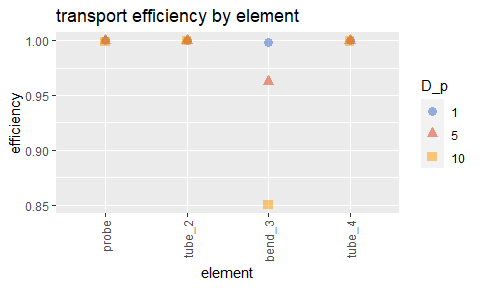report_cum_plots(df, 1)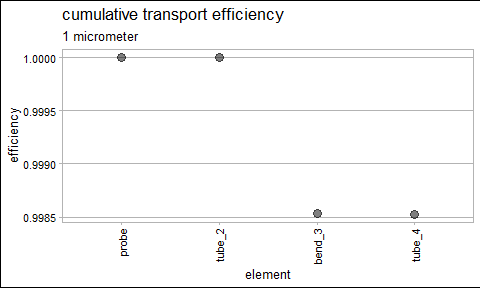report_cum_plots(df, 5)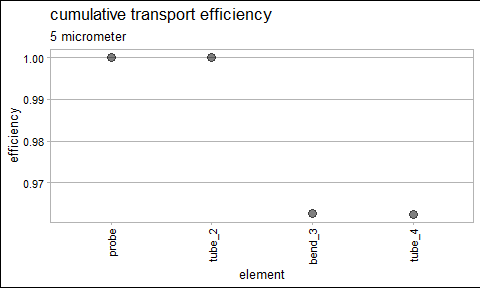report_cum_plots(df, 10)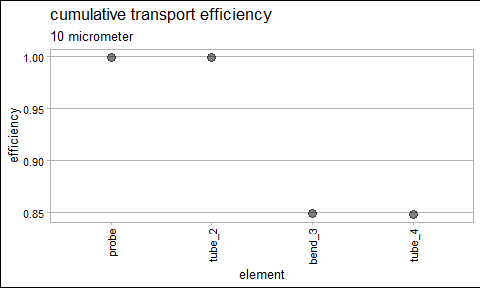ft <- flextable(report_basic(df, params, "log"))
#> System Parameters
#> All values in MKS units, except noted
#> Notes: D_tube is in m.
#> Q_lpm is system flow in liters per minute.
#> velocity_air is the derived air flow velocity in meters per second.T_K is system temperature in Kelvin.
#> P_kPa is system pressure in kiloPascals.
#> Re is the system Reynolds number, a measure of turbulence.
ft <- colformat_double(ft, digits = 3)
ft <- set_caption(ft, "results for log distribution of particle diameters")
ft  
results for log distribution of particle diameters

activity.fraction.sampled

0.170

### 6) Optional extra reports for the logarithmically distributed particle set

The report_log_mass function provides details on every particle size.

Since there are 1000 data points, the full ouptput is probably not suitable for a typical report. The report provides the following columns of output:

• microns = the particle size in micrometers (microns is shorter, but is considered supersed by micrometers)
• probs = relative probability of a particle being in this size bin
• bin_eff = the overall system efficiency for a particle of this size
• amb_mass = the probability of the particle multiplied by the mass of a spherical particle with the size given and density of 1 g per ml. This is the relative mass of this particle size in the ambient air being sampled.
• sampled_mass = the relative mass that made it through the sampling system with this particle size
• bin_frac_lost = ambient mass in this bin minus the sampled mass in the bin, divided by the ambient mass
• total_frac_lost = ambient mass in this bin minus the sampled mass in the bin, divided by the sum of the ambient mass

A random selection of ten rows of the 1000 rows are provided below:

df_log <- report_log_mass(df)[sort(sample(1:1000, 10)), ]
# need to make format changes so that flextable will show scientific notation
df_log[, 1] <- formatC(df_log[, 1], digits = 4)
df_log[, 2] <- formatC(df_log[, 2], digits = 2, format = "e")
df_log[, 3] <- formatC(df_log[, 3], digits = 2, format = "e")
df_log[, 4] <- formatC(df_log[, 4], digits = 2, format = "e")
df_log[, 5] <- formatC(df_log[, 5], digits = 2, format = "e")
df_log[, 6] <- formatC(df_log[, 6], digits = 2, format = "e")
df_log[, 7] <- formatC(df_log[, 7], digits = 2, format = "e")
ft <- flextable(df_log)
ft <- colformat_double(ft, digits = 3)
ft <- set_caption(ft, "results for random sample of 1000 particle diameters from the log set")
ft
results for random sample of 1000 particle diameters from the log set

D_p

dens

bin_eff

amb_mass

sampled_mass

bin_frac_lost

total_frac_lost

0.06161

7.09e-05

1.00e+00

6.49e-12

6.49e-12

5.74e-05

1.87e-19

0.1784

3.26e-03

1.00e+00

2.10e-08

2.10e-08

8.56e-05

9.02e-16

0.2015

4.65e-03

1.00e+00

4.88e-08

4.88e-08

9.93e-05

2.43e-15

0.4247

2.74e-02

1.00e+00

5.67e-06

5.67e-06

3.09e-04

8.78e-13

0.4858

3.52e-02

1.00e+00

1.25e-05

1.25e-05

3.89e-04

2.43e-12

1.101

1.01e-01

9.98e-01

9.47e-04

9.45e-04

1.78e-03

8.43e-10

15.8

1.25e-02

6.45e-01

4.97e+00

3.20e+00

3.55e-01

8.83e-04

17.64

9.58e-03

5.73e-01

5.90e+00

3.38e+00

4.27e-01

1.26e-03

44.65

5.62e-04

1.19e-02

1.42e+01

1.69e-01

9.88e-01

7.03e-03

87.42

3.80e-05

0.00e+00

1.41e+01

0.00e+00

1.00e+00

7.08e-03

The particle mass modeled in the ambient air and sampled through the air sampling system is shown with the function report_plots.

report_plots(df, "log")`